# fderivative

Derivative of a univariate, vector-valued function using the forward difference approximation.

## Syntax

df = fderivative(f,x0)
df = fderivative(f,x0,h)


## Description

df = fderivative(f,x0) numerically evaluates the derivative of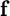with respect to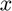at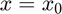using the forward difference approximation with a default relative step size of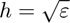, where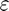is the machine zero.

df = fderivative(f,x0,h) numerically evaluates the derivative ofwith respect toatusing the forward difference approximation with a user-specified relative step size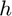.

## Input/Output Parameters

 Variable Symbol Description Format Input f univariate, vector-valued function () 1×1function_handle x0 evaluation point 1×1double h (OPTIONAL) relative step size 1×1double Output df derivative of with respect to , evaluated at m×1double

## Note

• This function requires 2 evaluations of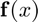.
• If the function is scalar-valued, then.

## Example #1: Derivative of a scalar-valued function.

Approximate the derivative of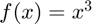at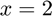using the fderivative function, and compare the result to the true result of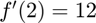.

Approximating the derivative,

f = @(x) x^3;
df = fderivative(f,2)

df =

12.0000



Calculating the error,

error = df-12

error =

2.7816e-07



## Example #2: Derivative of a vector-valued function.

Approximate the derivative of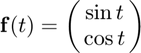at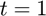using the fderivative function, and compare the result to the true result ofApproximating the derivative,

f = @(t) [sin(t);cos(t)];
df = fderivative(f,1)

df =

0.5403
-0.8415



Calculating the error,

error = df-[cos(1);-sin(1)]

error =

1.0e-07 *

-0.1436
-0.0925